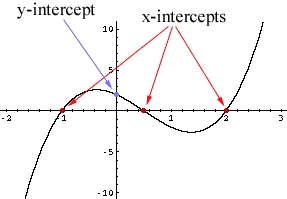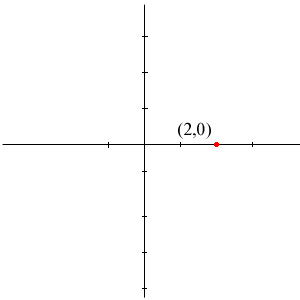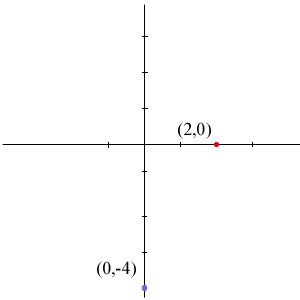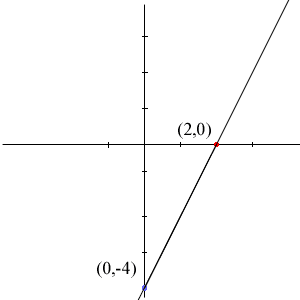Quandaries and Queries Hi. I am totally confused on how to determine intercepts of an equation and then to graph the line. For example, how to determine the intercepts of 2x-3y-12=0. I am a student in grade 10. If you could email me back that would be greatly appreciated. Thank you. Hi, The intercepts of a graph are the points where the graph crosses the axes. A point where the graph crosses the x-axis is called an x-intercept and a point where the graph crosses the y-axis is called a y-intercept.Points on the x-axis are points with y-cordinate equal to zero, that is they are points of the form (a,0) for some number a. Hence, for your equation 2x - 3y - 12 = 0 the x-intercepts are the points on the graph that have the y-cordinate equal to zero. To find them set y = 0 in the equation and solve for x. 2x - 3(0) -12 = 0 2x = 12 x = 2 Thus the x-interxept is the point (2,0)In a similar way the y-intercepts are points on the graph with coordinates x = 0. Thus let x = 0 and solve 2(0) - 3y - 12 = 0 giving y = -4 and hence a y-intercept of (0,-4).To draw the graph of this line,use a ruler and draw a line through (2,0) and (0,4).Penny Go to Math Central